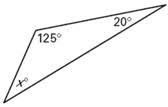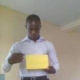# Mock Exam Questions – Second Term Examination Mathematics SS 3

Last Updated on May 24, 2021 by Alabi M. S.

SECOND TERM EXAMINATION

MOCK EXAMINATION

SENIOR SECONDARY SCHOOL (SS 3)

### MATHEMATICS

SECTION A – OBJECTIVES

1. Express the product of 0.06 and 0.09 in standard FORM.

a. 5.4 x 10¯³

b. 5.4 x 10¯²

c. 5.4 x 10¯¹

d. 5.4 x 10²

2. Simplify (27 1/3)²

a. 4 ½

b. 6

c. 9

d. 18

Using the table below for question 3 to 5.

Score                0    1    2    3    4    5

Frequency        2    3    4    2    7    2

3. Find the mode

a. 2

b. 3

c. 4

d. 5

4. Find the mean score

a. 0

b. 2.5

c. 3

d. 5

5. The median score is _________.

a. 0

b. 2.5

c. 3

d. 5

6. What is the probability of having an odd number in a single toss of a fair dice?

a. 1/6

b. 1/3

c. ½

d. 2/3

7. Evaluate log6 + log45 – log27

a. 0

b. 1

c. 1.1738

d. 1.3802

8. Factorize 5y2 + 2ay – 3a2

a. (5y-a)(y+3a)

b. (5y+a)(y-3a)

c. 5y2+a(2y-3a)

d. (y+a)(5y-3a)

9. What value of K makes the given expression a perfect square? M2 – 8m + k

a. 2

b. 4

c. 8

d. 16

10. if log10A = 4; what is A?

a. 0.410

b. 40

c. 400

d. 100

11. Find the area of triangle in fig 1.a. 30 cm²

b. 30 cm³

C. 30 cm¹

D. 30 cm

12. If the 2nd and 4th terms of a G. P. are 8 and 32 respectively, what is the sum of the 1st 4 terms.

a. 28

b. 40

c. 48

d. 60

13. Find the volume of a cone of radius 3.5cm and vertical height 12cm. π=22/7

a. 3.4 cm³

b. 15.5 cm³

c. 21.0 cm³

d. 42 cm³

14. The angle of a sector of a circle of diameter 8cm is 135o. Find the area of the sector.

a. 9 3/7 cm²

b. 12 4/7 cm²

c. 12 4/7 cm²

d. 25 1/7 cm²

15. If sin θ =1/2 and Cos θ = -√3/2, what is the value of θ?

a. 30º

b. 60º

c. 90º

d. 120º

16. Solve for t in equation ¾ t + 1/3 (21-t) = 11

a. 9/13

b. 9/

c. 5

d. 9 3/5

17. A school girl spends ¼ of her pocket money on books and 1/3 on dress. What fraction remains?

a. 5/6

b. 7/12

c. 5/12

d. 1/6

18. Find x, using fig 2.a. 18º

b. 28º

c. 35º

d. 44º

19. If logqp = r, express p in terms of q and r.

a. p = qr

b. p = rq

c. p = r/q

d. p = qr

20. Given that √128 + √18 – √k = 7√2, find k.

a. 8

b. 16

c. 32

d. 48

21. Divide #900 in the ratio 3 : 2

a. 540 : 300

b. 360 : 540

c. 540 : 360

d. 500 : 400

22. Factorize x² – 8x + 16 = 16.

a. (x+4) (x+4)

b. (x+4) (x-4)

c. (x-4) (x-4)

d. (x-4) (x+4)

23. In the below triangle, x is a ___________ angle.a. reflex

b. obtuse

c. right

d. acute

24. Express 0.006313 in a standard form.

a. 63.13 x 10²

b. 6.313 x 10¯³

c. 63.13 x 10¯²

d. 6.313 x 10³

25. A point X is 34m due east of a point Y, the bearing of a flagpole from X and Y are N18º W and N40º E respectively. Calculate the distance of the flagpole Y.

a. 40.7 m

b. 30 m

c. 30.7 m

d. 40 m

26. Fig 4 means

a. y < -2

b. y ≤ -2

c. y > -2

d. y ≥ -2

27. Find a, when A = 58.1o, b = 10m, c = 8.5m

a. 9.5m

b. 9.4m

c. 89.8 m

d. 8.98 m

28. Express 6700 in a standard form.

a. 6.7 x 10¯³

b. 6.7 x 10³

c. 6.7 x 10¯²

d. 6.7 x 10¯²

29. Solve for x in equation 2×3 = 54

a. 4

b. 27

c. 3

d. 1/3

30. Evaluate 3.802 x 2.09 using rough check.

a. 8

b. 7.95

c. 8.95

d.7

31. Convert 210three to base ten.

a. 21ten

b. 20ten

c. 22ten

d. 210ten

32. Simplify 5/2 – x/2 + 5 to a single fraction.

a. (3 – x) + 10/2

B. (7 – x)/2

c. (13 – x)/4

d. (13 – x)/2

b. 3a(b + c + d)

c. 1ab(3 + 6 + 3)

d. 3a(b – 2c + d)

34. a triangle has sides 8cm and 5cm and angles of 90o between them. Calculate the small angle.

a. 58.3º

b. 32.1º

c. 90º

d. 45º

35. Convert 110101two to base ten.

a. 53ten

b. 49ten

c. 55ten

d. 32ten

36. When x = 3, y = 5. What will x be when y =25

a. 15

b. 23

c. 20

d. 27

37. In triangle ABC, C = 53o , b = 3.56m, c = 4.28m. Calculate B.

a. 41º

b. 41.5º

c. 40º

d. 42º

38. Q = Q3-Q1/2 defines ____________.

a. quartile

b. semi quartile

c. semi inter quartile range

d. inter quartile range

Using fig 5 to answer 39-40.

39. Find x

a. 2

b. 14

c. 28

d. 48

40. How many students are in group B?

a. 16

b. 30

c. 18

d. 42

41. In a class of 35 students, 19 take history and 12 take economics. If 5 take both subjects, how many take neither?

a. 7

b. 8

c. 6

d. 5

42.

a. x < -5

b. x ≤ -5

c. x > -5

d. x ≥ -5

43. Reduce 360x2y/300xy2 to the lowest term.

a. 6y/5x

b. 6x/5y

c. 5x/6y

d. 5y/6x

44. Which of the statement is correct about alternating angle?

a. a = b

b. a = p

c. a = q

d. none of the above.

45. Calculate a, using triangle ABC, A = 29º, B=36º, b=15.8cm

a. 13

b. 13.1

c. 14

d. 14.1

Using the pie chart fig 6 to answer number 46-49.

46. What fraction of the time is spent sleeping?

a. 3/8

b. ¼

c. 2/5

d. 8/24

47. What percentage of the time is spent studying?

a. 50%

b. 2.5%

c. 25%

d.33.3%

48. How much time is spent studying?

a. 3 hrs

b. 4 hrs

c. 4 hrs 30mins

d. 5hrs

49. How much time is spent travelling, calc. the value of x.

a. 87ª

b. 24º

c. 48o

d. 12º

50. Calculate the volume of cone of fig 7.

a. 3,193.5 cm³

b. 4,714.3 cm³

c. 4,714.2 cm³

d. 3,193.4 cm³

### SECTION B – THEORY

Question 1

a. Solve 1 = 27(1-x) 81(x-2)

b. Simplify 5/√7-√3 + 1/√7 – √3 leaving your answer in surd form.

Question 2

The diagram fig 1 shows the position of 3 points X,Y,Z on the horizontal plane. The bearing of Y from X is 312o and that of Y from Z is 022o. if /XY/ = 32 km and /ZY/ = 50km. calculate, correct to one decimal place.

i. /XY/

ii. The bearing of Z from X

Question 3

CLASS INTERVAL              FREQUENCY

60  –  64                                   2

65  –  69                                   3

70  –  74                                   6

75  –  79                                  11

80  –  84                                   8

85  –  89                                   7

90  –  94                                   2

95  –  99                                   1

Calculate the

a. Mean

b. Standard deviation of the distribution in 2 d.p.

Question 4

a. Two positive whole number P and Q are such that P is greater than Q and their sum is equal to 3 times their difference, express P in terms of Q

b. Hence, evaluate

I. P2 + Q2

II. PQ

1.•